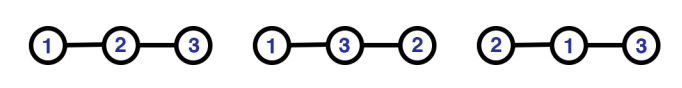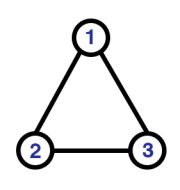시간 제한 메모리 제한 제출 정답 맞은 사람 정답 비율
2 초 512 MB 0 0 0 0.000%

## 문제

First, let’s define an undirected connected labeled graph, it’s a graph with N nodes with a unique label for each node and some edges, there’s no specific direction for each edge, also duplicate edges and edges from a node to itself aren’t allowed, and from any node you can reach any other node.

A bridge in such graph is an edge that if we remove it, the graph will be disconnected (there will exist nodes which aren’t reachable from each other).

In this problem you are given N and K, and your task is to count the number of different undirected connected labeled graphs with exactly N nodes and K bridges. Since that number can be huge, print it modulo M.

An edge is defined using the labels of the nodes it connects, for example we can say (X, Y ) is an edge between X and Y , also (Y, X) is considered the same edge (since it’s undirected). Two graphs are considered different, if there’s an edge which exists in one of them but not the other.

## 입력

Your program will be tested on one or more test cases. The first line of the input will be a single integer T (1 ≤ T ≤ 100) representing the number of test cases. Followed by T test cases.

Each test case will be just one line containing 3 integers separated by a space, N (1 ≤ N ≤ 50), K (0 ≤ K < N) and M (1 ≤ M ≤ 109), which are the numbers described in the statement.

It’s guaranteed that N will not be more than 25 in 95% of the test cases.

## 출력

For each test case, print a single line with the number of graphs as described above modulo M.

## 예제 입력 1

4
3 2 10
3 0 10
6 3 10000
6 3 1000


## 예제 출력 1

3
1
2160
160


## 힌트

The following are the 3 graphs for the first test case:The following is the only graph for the second test case: